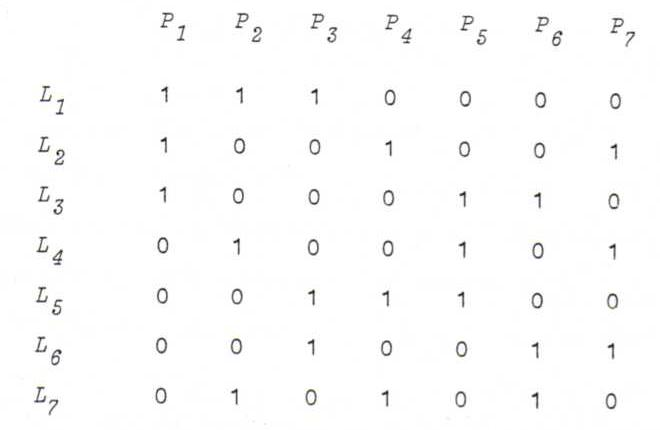### COURSE OF PROJECTIVE GEOMETRY

O5 Let p be a straight line through O.
If p is lying in x3=0, we associate p with the point at infinity in x3=1 in the same direction.
If p is not lying in x3=0, we associate p with the point where it intersects x3=1.

Let l be a plane through O.
If l is the plane x3=0, we associate l with the line at infinity.
If l is some other plane, we associate l with its line of intersection with x3=1.

Thereafter, define the incidence relation in (P",L") using these bijections: so Ap is incident with Bl if and only if p is incident with l.

O6 Map each straight line through O to its point of intersection with B, and each plane through O to its circle of intersection with B.
This yields a bijection φ:PP''' and a bijection ψ:LL''' so that φ(p) is incident with ψ(l) if and only if p is incident with l.

O7If we call L the set of points and P the set of lines, we get the dual geometry, which is isomorphic to the original one.
Last question:
Every projective geometry contains at least seven points, because:
i) P is not empty, so there is a point p1.
ii) p1 is lying on at least three distinct lines l1, l2, l3 (axiom A2).
iii) On l1 there are at least three points p1, p2, p3 (axiom A1).
iv) On l2 there are two points p4 and p5 that don't coincide with p1, p2 or p3 (A1, and A3 or A4).
v) Likewise, on l3 there are two points p6 and p7 that don't coincide with any of the five other points.